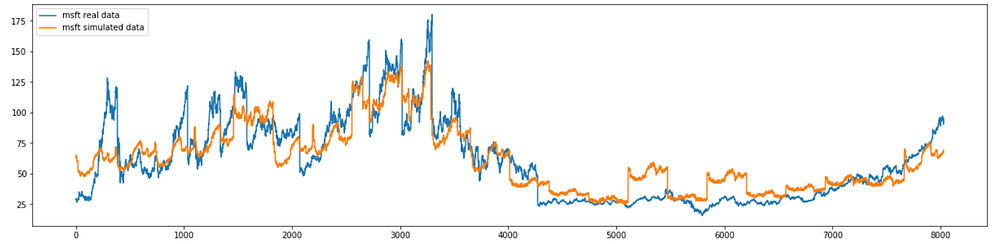# Generating Simulated Stock Price Data using a Variational Autoencoder

Source: Deep Learning on Medium# Generating Simulated Stock Price Data using a Variational Autoencoder

Standard autoencoders, which are useful for tasks like data compression and denoising, learn from training data in order to generate a compact representation of the original input. Variational autoencoders, on the other hand, are powerful generative models that generate new data that looks similar to the training data.

A very good and intuitive explanation of the principles behind variational autoencoders can be found here.

A common requirement in software development is to generate simulated data for the development work, so that potentially sensitive information is not exposed to the development teams. This is especially true when working with financial data: if real-life data is not available to the development teams, they will at least need simulated datasets whose behavior looks like real data.

In this example, I will use a simple variational autoencoder to simulate the stock price of three technological companies: Microsoft (msft), Apple (aapl) and Amazon (amzn). I used data publicly available in Quandl to train the model, reserving the last 365 days for testing. The following is the Python code to retrieve this data:

`!pip install — upgrade tensorflow!pip install quandlimport tensorflow as tffrom tensorflow import kerasimport matplotlib.pyplot as pltimport numpy as npfrom sklearn import preprocessingfrom sklearn.model_selection import train_test_splitimport quandlquandl_data_apple = quandl.get(‘WIKI/AAPL’)quandl_data_amazon = quandl.get(‘WIKI/AMZN’)quandl_data_msft = quandl.get(‘WIKI/MSFT’)`

The training data (which is normalized before going into the model) is constituted by chunks of 365 consecutive data points corresponding to the highest stock price of the day (I am not using the adjusted price in this model, as the goal of this example is not prediction but data generation). The last 365 days are reserved for testing:

`msft_high_raw = (quandl_data_msft['High'].values).reshape(1,-1)msft_high = preprocessing.normalize(msft_high_raw, norm='max', axis=1)msft_high = msft_high.reshape(msft_high.shape,)msft_max_high = np.max(msft_high_raw)apple_high_raw = (quandl_data_apple['High'].values).reshape(1,-1)apple_high = preprocessing.normalize(apple_high_raw, norm='max', axis=1)apple_high = apple_high.reshape(apple_high.shape,)apple_max_high = np.max(apple_high_raw)amazon_high_raw = (quandl_data_amazon['High'].values).reshape(1,-1)amazon_high = preprocessing.normalize(amazon_high_raw, norm='max', axis=1)amazon_high = amazon_high.reshape(amazon_high.shape,)amazon_max_high = np.max(amazon_high_raw)def generate_samples(data, sample_size): n_samples = data.shape//sample_size result = np.empty((n_samples, sample_size)) for i in range(n_samples): result[i] = data[i*sample_size : i*sample_size + sample_size] return(result)test_data_points = 365sample_size = 365msft_high_train = msft_high[0:msft_high.shape-test_data_points]msft_high_test = msft_high[-test_data_points:]X_msft_train = generate_samples(msft_high_train, sample_size)X_msft_test = generate_samples(msft_high_test, sample_size)apple_high_train = apple_high[0:apple_high.shape-test_data_points]apple_high_test = apple_high[-test_data_points:]X_apple_train = generate_samples(apple_high_train, sample_size)X_apple_test = generate_samples(apple_high_test, sample_size)amazon_high_train = amazon_high[0:amazon_high.shape-test_data_points]amazon_high_test = amazon_high[-test_data_points:]X_amazon_train = generate_samples(amazon_high_train, sample_size)X_amazon_test = generate_samples(amazon_high_test, sample_size)`

The autoencoder has the following architecture:

• Encoder network: 4 dense layers of size 256, 128, 32 and 16, with 0.1 dropout after the first two layers. The hidden layers use relu activation.
• Decoder network: 4 dense layers of size 16, 32, 128 and 256. The hidden layers use relu activation and the output layer uses tanh activation.
• 1-Dimensional latent space.